Students can download Maths Chapter 3 Algebra Ex 3.12 Questions and Answers, Notes, Samacheer Kalvi 10th Maths Guide Pdf helps you to revise the complete Tamilnadu State Board New Syllabus, helps students complete homework assignments and to score high marks in board exams.

## Tamilnadu Samacheer Kalvi 10th Maths Solutions Chapter 3 Algebra Ex 3.12

Question 1.
If the difference between a number and its reciprocal is $$\frac { 24 }{ 5 }$$, find the number.
Let the number be “x” and its reciprocal is $$\frac { 1 }{ x }$$
By the given condition
x – $$\frac { 1 }{ x }$$ = $$\frac { 24 }{ 5 }$$
$$\frac{x^{2}-1}{x}$$ = $$\frac { 24 }{ 5 }$$
5x2 – 5 = 24x
5x2 – 24x – 5 = 0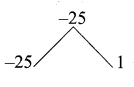5x2 – 25x + x – 5 = 0 ⇒ 5x (x – 5) + 1(x – 5) = 0
(x – 5) (5x – 1) = 0 ⇒ x – 5 = 0 or 5x + 1 = 0
x = 5 or 5x = -1 ⇒ x = $$\frac { -1 }{ 5 }$$
The number is 5 or $$\frac { -1 }{ 5 }$$Question 2.
A garden measuring 12m by 16m is to have a pedestrian pathway that is meters wide installed all the way around so that it increases the total area to 285 m2. What is the width of the pathway?
Let the width of the rectangle be “ω”
Length of the outer rectangle = 16 + (ω + ω)
16 + 2ω
Breadth of the outer rectangle = 12 + 2ω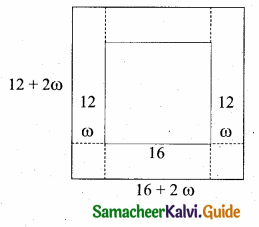By the given condition
(16 + 2ω) (12 + 2ω) = 285
192 + 32 ω + 24 ω + 4 ω2 = 285
4 ω2 + 56ω = 285 – 192
4 ω2 + 56 ω = 93
4 ω2+ 56 ω – 93 = 0
Here a = 4, b = 56, c = -93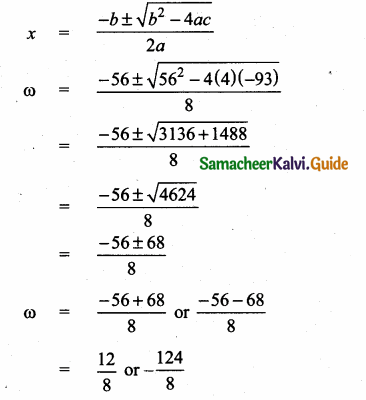= 1.5 or -15.5 (Width is not negative)
∴ Width of the path way = 1.5 mQuestion 3.
A bus covers a distance of 90 km at a uniform speed. Had the speed been 15 km/hour more it would have taken 30 minutes less for the journey. Find the original speed of the journey.
Let the original speed of the bus be “x” km/hr
Time taken to cover 90 km = $$\frac { 90 }{ x }$$
After increasing the speed by 15 km/hr
Time taken to cover 90 km = $$\frac { 90 }{ x+15 }$$
By the given condition
$$\frac { 90 }{ x }$$ – $$\frac { 90 }{ x+15 }$$ = $$\frac { 1 }{ 2 }$$
$$\frac{90(x+15)-90 x}{x(x+15)}$$ = $$\frac { 1 }{ 2 }$$
90x + 1350 – 90x = $$\frac{x^{2}+15 x}{2}$$
1350 = $$\frac{x^{2}+15 x}{2}$$
2700 = x2 + 15x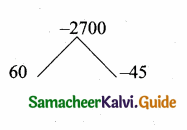x2 + 15x – 2700 = 0
(x + 60) (x – 45) = 0
x + 60 = 0 or x – 45 = 0
x = – 60 or x = 45
The speed will not be negative
∴ Original speed of the bus = 45 km/hrQuestion 4.
A girl is twice as old as her sister. Five years hence, the product of their ages – (in years) will be 375. Find their present ages.
Let the age of the sister be “x”
The age of the girl = 2x
Five years hence
Age of the sister = x + 5
Age of the girl = 2x + 5
By the given condition
(x + 5) (2x + 5) = 375
2x2 + 5x + 10x + 25 = 375
2x2 + 15x – 350 = 0
a = 2, b = 15, c = -350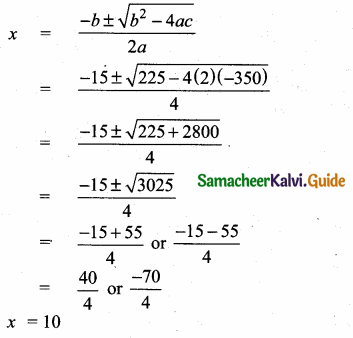Age will not be negative
Age of the girl = 10 years
Age of the sister = 20 years (2 × 10)Question 5.
A pole has to be erected at a point on the boundary of a circular ground of diameter 20 m in such a way that the difference of its distances from two diametricallyopposite fixed gates P and Q on the boundary is 4 m. Is it possible to do so? If answer is yes at what distance from the two gates should the pole be erected?
Let “R” be the required location of the pole
Let the distance from the gate P is “x” m : PR = “x” m
The distance from the gate Q is (x + 4)m
∴ QR = (x + 4)m
In the right ∆ PQR,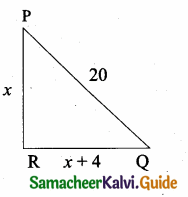PR2 + QR2 = PQ2 (By Pythagoras theorem)
x2 + (x + 4)2 = 202
x2 + x2 + 16 + 8x = 400
2x2 + 8x – 384 = 0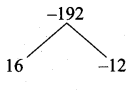x2 + 4x – 192 = 0(divided by 2)
(x + 16) (x – 12) = 0
x + 16 = 0 or x – 12 = 0 [negative value is not considered]
x = -16 or x = 12
Yes it is possible to erect
The distance from the two gates are 12 m and 16 mQuestion 6.
From a group of 2x2 black bees , square root of half of the group went to a tree. Again eight-ninth of the bees went to the same tree. The remaining two got caught up in a fragrant lotus. How many bees were there in total?
Total numbers of black bees = 2x2
Half of the group = $$\frac { 1 }{ 2 }$$ × 2x2 = x2
Square root of half of the group = $$\sqrt{x^{2}}$$ = x
Eight – ninth of the bees = $$\frac { 8 }{ 9 }$$ × 2x2 = $$\frac{16 x^{2}}{9}$$
Number ofbees in the lotus = 2
By the given condition
x + $$\frac{16 x^{2}}{9}$$ + 2 = 2x2
(Multiply by 9) 9x + 16×2 + 18 = 18x2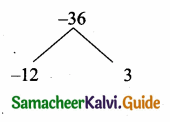18x2 – 16x2 – 9x – 18 = 0 ⇒ 2x2 – 9x – 18 = 0
2x2 – 12x + 3x – 18 = 0
2x(x – 6) + 3 (x – 6) = 0
(x – 6) (2x + 3) = 0
x – 6 = 0 or 2x + 3 = 0
x = 6 or 2x = -3 ⇒ x = $$\frac { -3 }{ 2 }$$ (number of bees will not be negative)
Total number of black bees = 2x2 = 2(6)2
= 72Question 7.
Music is been played in two opposite galleries with certain group of people. In the first gallery a group of 4 singers were singing and in the second gallery 9 singers were singing. The two galleries are separated by the distance of 70 m. Where should a person stand for hearing the same intensity of the singers voice?
(Hint: The ratio of the sound intensity is equal to the square of the ratio of their corresponding distances).
Number of singers in the first group = 4
Number of singers in the second group = 9
Distance between the two galleries = 70 m
Let the distance of the person from the first group be x
and the distance of the person from the second group be 70 – x
By the given condition
4 : 9 = x2 : (70 – x)2 (by the given hint)
$$\frac { 4 }{ 9 }$$ = $$\frac{x^{2}}{(70-x)^{2}}$$
$$\frac { 2 }{ 3 }$$ = $$\frac { x }{ 70-x }$$ [taking square root on both sides]
3x = 140 – 2x
5x = 140
x = $$\frac { 140 }{ 5 }$$ = 28
The required distance to hear same intensity of the singers voice from the first galleries is 28m
The required distance to hear same intensity of the singers voice from the second galleries is (70 – 28) = 42 mQuestion 8.
There is a square field whose side is 10 m. A square flower bed is prepared in its centre leaving a gravel path all round the flower bed. The total cost of laying the flower bed and gravelling the path at ₹3 and ₹4 per square metre respectively is ₹364. Find the width of the gravel path.
Let the width of the gravel path be ‘x’
Side of the flower bed = 10 – (x + x)
= 10 – 2x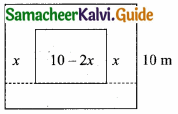Area of the path way = Area of the field – Area of the flower bed
= 10 × 10 – (10 – 2x) (10 – 2x) sq.m
= 100 – (100 + 4x2 – 40x)
= 100 – 100 – 4x2 + 40x
= 40x – 4x2 sq.m
Area of the flower bed = (10 – 2x) (10 – 2x) sq.m.
= 100 + 4x2 – 40x
By the given condition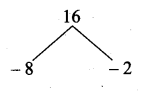3(100 + 4x2 – 40x) + 4(40x – 4x2) = 364
300 + 12x2 – 120x + 160x – 16x2 = 364
-4x2 + 40x + 300 – 364 = 0
-4x2 + 40x – 64 = 0
(÷ by 4) ⇒ x2 – 10x + 16 = 0
[The width must not be equal to 8 m since the side of the field is 10m]
(x – 8) (x – 2) = 0
x – 8 = 0 or x – 2 = 0 x = 8 or x = 2
Width of the gravel path = 2 mQuestion 9.
Two women together took 100 eggs to a market, one had more than the other. Both sold them for the same sum of money. The first then said to the second: “If I had your eggs, I would have earned ₹ 15”, to which the second replied: “If I had your eggs, I would have earned ₹ 6 $$\frac{2}{3}$$ How many eggs did each had in the beginning?
Solution:
Let the no. of eggs with woman 1 be x and woman 2 be y.
∴ x + y = 100
Let w1 sell the eggs at ₹ ‘a’ per egg.
Let w2 sell the eggs at ₹ ‘b’ per egg.
Case 1:
They sold them for same money.
∴ ax = by
Case 2:
ay = 15 and bx = $$\frac{20}{3}$$
∴ One woman had 40 eggs and the other had 60 eggs.Question 10.
The hypotenuse of a right angled triangle is 25 cm and its perimeter 56 cm. Find the length of the smallest side.
Perimeter of a right angle triangle = 56 cm
Sum of the two sides + hypotenuse = 56
Sum of the two sides = 56 – 25
= 31 cm
Let one side of the triangle be “x”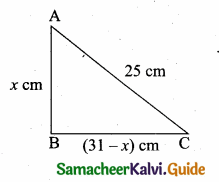The other side of the triangle = (31 – x) cm
By Pythagoras theorem
AB2 + BC2 = AC2
x2 + (31 – x)2 = 252
x2 + 961 + x2 – 62x = 625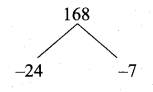2x2 – 62x + 961 – 625 = 0
2x2 – 62x + 336 = 0 ⇒ x2 – 31x + 168 = 0
(x – 24) (x – 7) = 0
x – 24 = 0 (or) x – 7 = 0
x = 24 (or) x = 7
Length of the smallest side is 7 cm